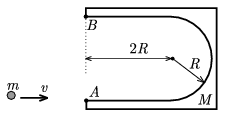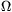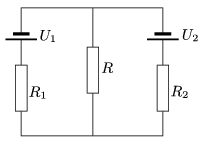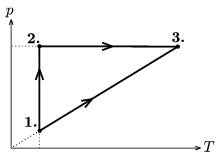Mathematical and Physical Journal
for High Schools
Issued by the MATFUND Foundation
 Already signed up? New to KöMaL?

# KöMaL Problems in Physics, December 2006

Show/hide problems of signs:## Problems with sign 'M'

Deadline expired on January 11, 2007.

M. 274. Measure how the torque exerted by a clothes peg depends on the angle between the prongs of a clothes peg. How much mechanical work is to be done to open the prongs of the peg completely.

(6 pont)

statistics## Problems with sign 'P'

Deadline expired on January 11, 2007.

P. 3933. A wheel, which has five spokes, turns 1200 in every minute. It is illuminated by a fluorescent lamp operated from a mains electricity supply. What is its apparent number of turns?

(3 pont)

statistics

P. 3934. The plates of a parallel plate condenser are horizontal and a small charged oil drop is floating between them. In what direction will the drop move if the plates of the condenser, which are connected to a battery which supplies constant voltage, are moved closer to each other?

(3 pont)

statistics

P. 3935. A piece of ice has a shape of a cone and it floats in water, such that its symmetry axis is vertical. What fraction of its height is above the water?

(4 pont)

statistics

P. 3936. Observing from the Earth by what factor does the diameter of the Moon seem to be greater than that of the Mars?

(4 pont)

statistics

P. 3937. Two wagons of masses m1 and m2 are moving towards each other along a horizontal path. Their speeds are v1 and v2 respectively. a) What is their common velocity after they are hitched? b) What is the maximum compression of each buffer if the spring constant of all the four buffers are the same D? c) If the sum of the masses of the two wagons is constant at what ratio of the masses will the maximum compression be the greatest?

(5 pont)

statistics

P. 3938. The U-shaped body of mass M shown in the figure is at rest and lies on a smooth horizontal surface (e.g. on ice). Its centre of mass is the midpoint of the semicircle of radius R. The length of the straight arms of the U is 2R. A small disc of mass m slides tangentially to point A on one of the arms, with a velocity of v.a) Where will point A be when the disc is at point B on the other arm?

b) Draw the path of the two bodies.

c) Find the velocity versus time and acceleration versus time functions for both bodies.

(Friction and air drag are negligible everywhere. Data: M=m=1 kg, R=0.1 m, v=0.5 m/s.)

(5 pont)

statistics

P. 3939. Two 100-resistors are connected in series, and they are operated from a supply which generates constant voltage. A variable resistor is connected to one of the 100-resistors in parallel. Find the resistance of the variable resistor when it dissipates the greatest power.

(4 pont)

statistics

P. 3940. Two voltage supplies are connected parallel to the same resistor. The electromotive force of one of them is U1, and its internal resistance is R1. The emf of the other is U2, and its internal resistance is R2. What is the resistance R of the resistor if it is to dissipate the greatest power?(4 pont)

statistics

P. 3941. A diatomic ideal gas is taken through the cyclic process shown in the figure. In state 1 the pressure of the gas is 100 kPa and its temperature is 350 K. In state 2 the pressure is 300 kPa. What is the efficiency of the cycle?(5 pont)

statistics

P. 3942. A lab animal is injected with a solution which contains a radioactive isotope of half-life 15 h. The activity of the solution is 2100 Bq. 5  hours later a sample of blood is taken from the animal and it was found that the activity of 3 cm3 of the animal's blood is 0.25 Bq. How many liters of blood does the animal have if during the elapsed five hours half of the solution was absorbed by its body?

(4 pont)

statistics